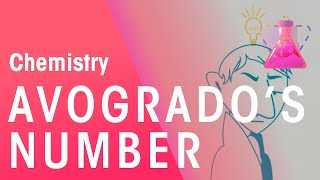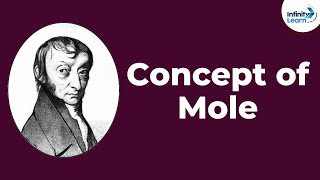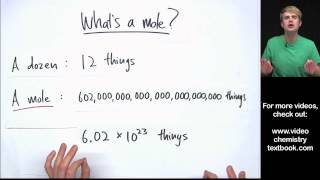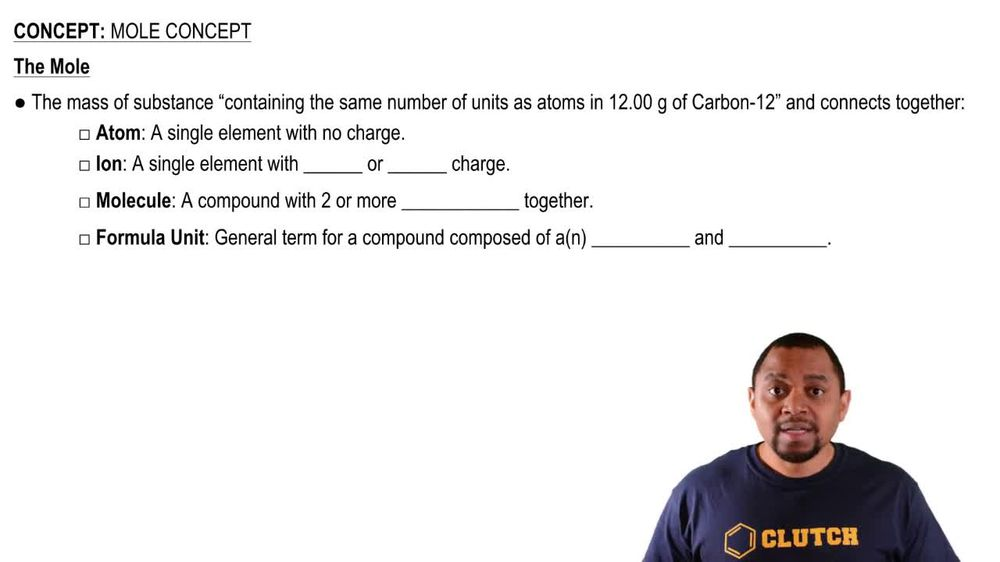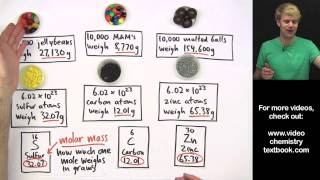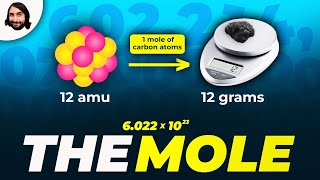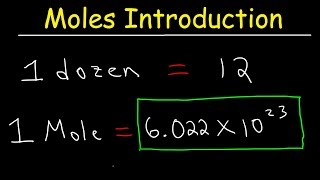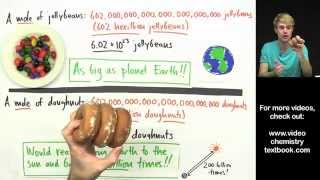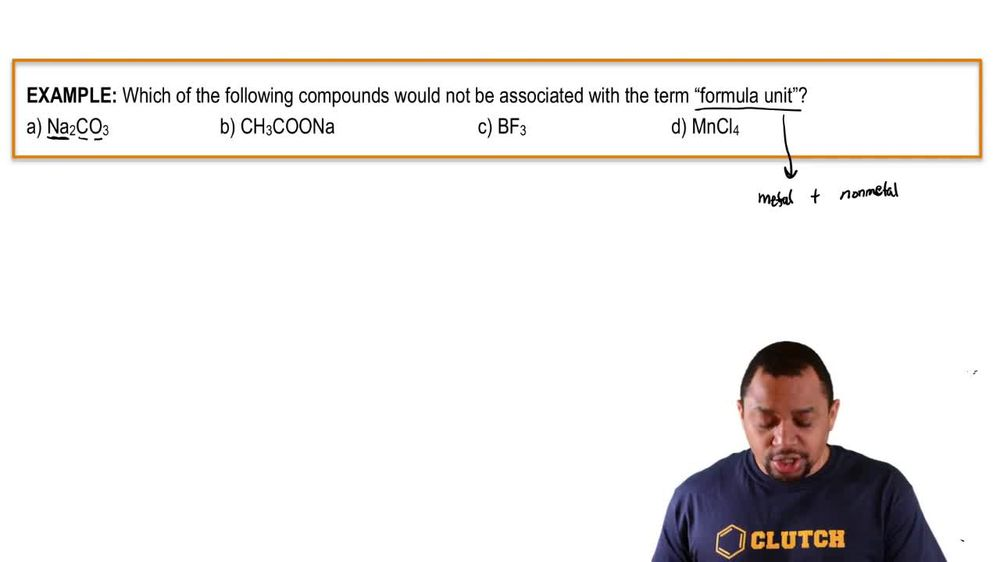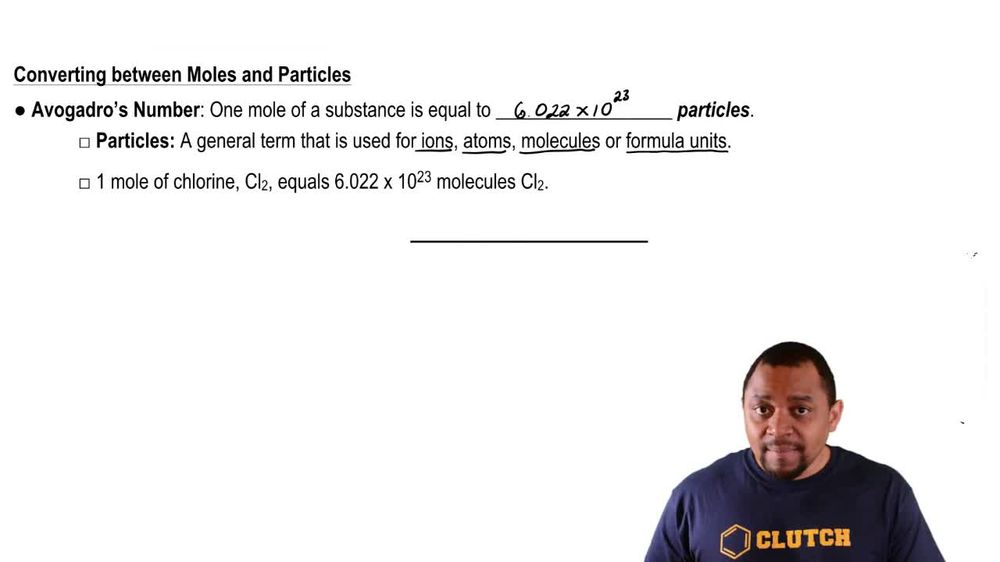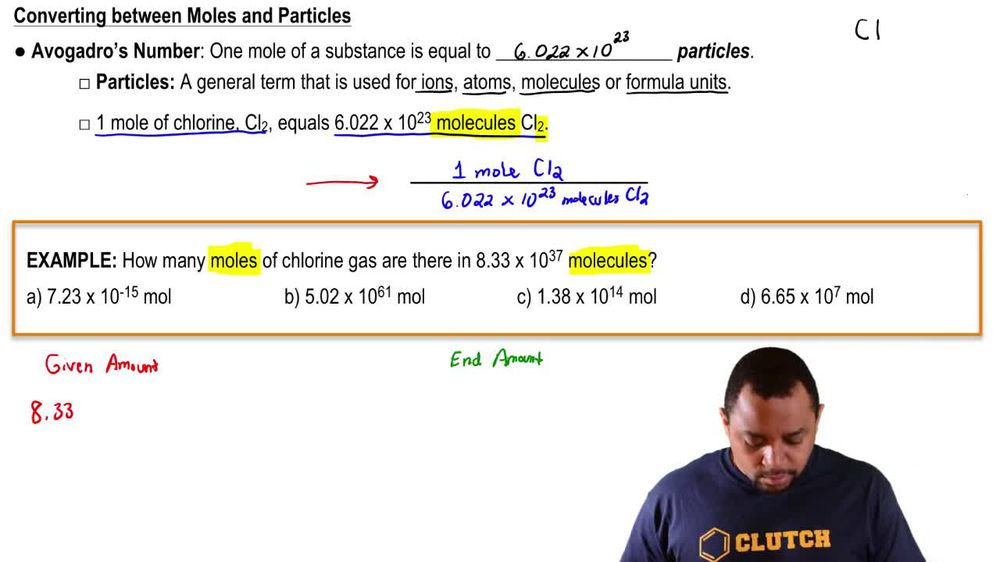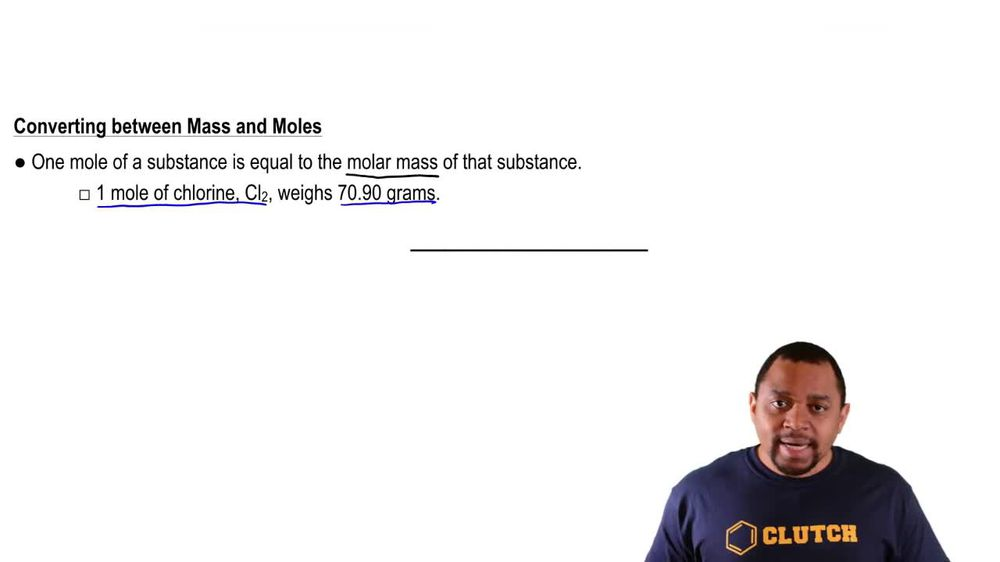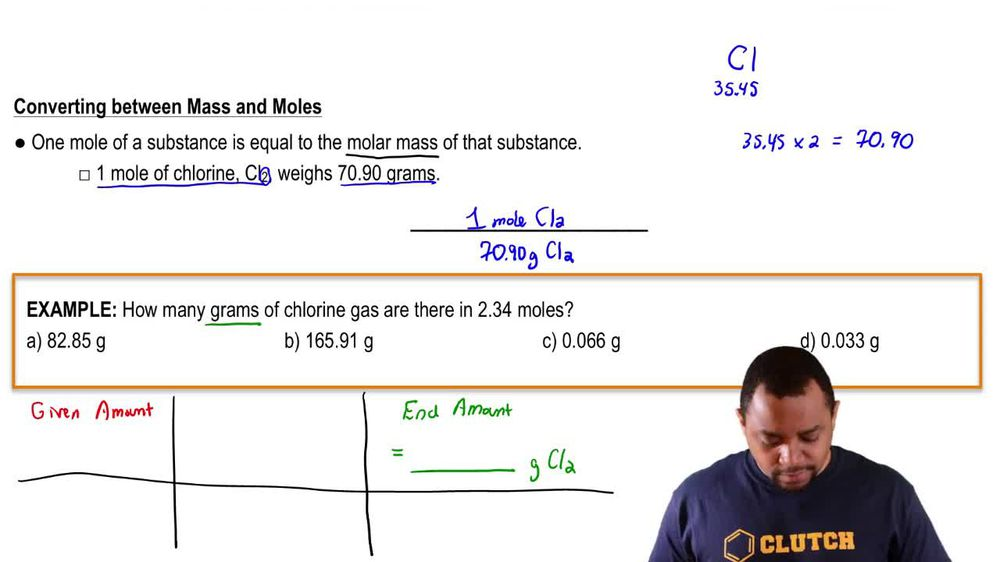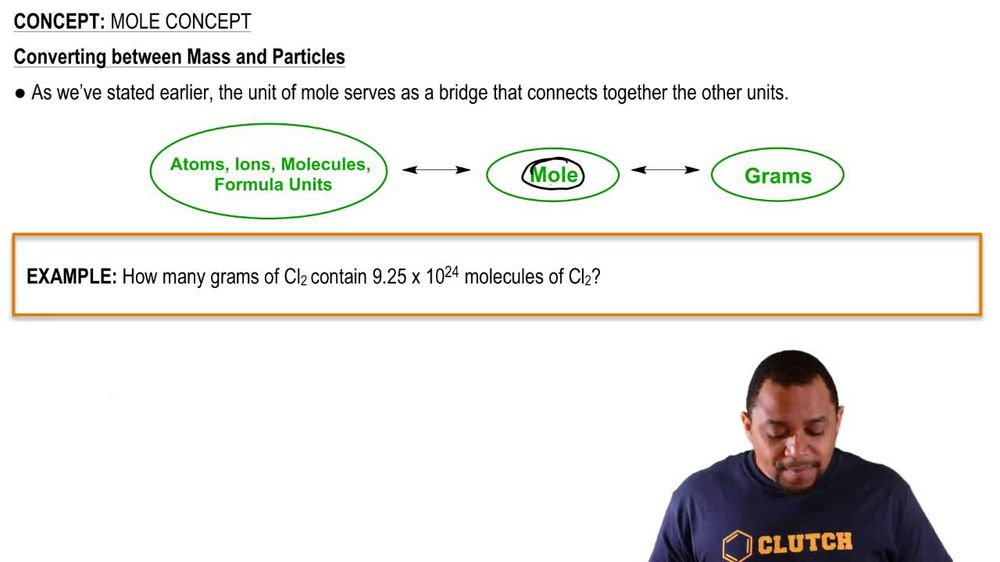Start typing, then use the up and down arrows to select an option from the list.
1. 2. Atoms & Elements2. Mole Concept
Problem

# How many helium atoms are there in a helium blimp containing 536 kg of helium?

Relevant Solution1m
Play a video:
Hey everyone, we're asked to calculate the number of gold atoms in a mg pendant of pure gold. We're first going to need to obtain the molar mass of gold since we're going to use that value in our dimensional analysis. Looking at our periodic table, We find that we have 196 . g of gold per one mole of gold. So starting off with our 125 mg of gold, we're going to convert our milligrams into grams. So we know that we have 10 to the third milligrams per one g. And now we're going to take our molar mass of 196.96 g per one mole. Now, since we want to go to Adams, we're going to take our one mole of gold and we're going to use avocados number and we know that per one mole, we have 6.22 times 10 to the 23rd gold atoms. So when we calculate this out and cancel out our units, we end up with a total of 3.8, 2 times 10 to the atoms of gold. And this is going to be our final answer. So I hope this made sense. And let us know if you have any questions